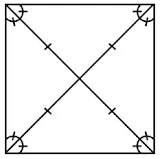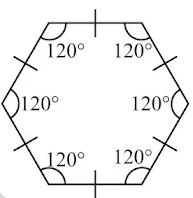# Chapter 14 Symmetry Class 7 Notes Maths

Chapter 14 Symmetry Class 7 Notes Maths is available here which will make entire memorizing process effortless and entertaining. NCERT Notes becomes a vital resource for all the students to self-study from NCERT textbooks carefully. Revision Notes for Class 7 will allows the students to evaluate their learning immediately. A student will enjoy the revising process and make themselves capable of retaining more information so they can excel in the exams. You will find NCERT Solutions for Class 7 Chapter 14 Maths that can make things a little easier for you.Line Symmetry

• A figure has a line symmetry, if there is a line about which the figure may be folded so that the two parts of the figure will coincide.

Lines of symmetry for regular polygons

• A polygon is said to be regular if all its sides are of equal length and all its angles are of equal measure.

• An equilateral triangle is regular because each of its sides has same length and each of its angles measures 60°.• A square is also regular because all its sides are of equal length and each of its angles is a right angle (i.e., 90°). Its diagonals are seen to be perpendicular bisectors of one another.• If a pentagon is regular, naturally, its sides should have equal length. The measure of each of its angles is 108°.• A regular hexagon has all its sides equal and each of its angles measures 120°.Rotational symmetry

• Rotation turns an object about a fixed point. This fixed point is the centre of rotation. The angle by which the object rotates is the angle of rotation.

• A half-turn means rotation by 180°; a quarter-turn means rotation by 90°. Rotation may be clockwise or anticlockwise.

• If, after a rotation, an object looks exactly the same, we say that it has a rotational symmetry.

• In a complete turn (of 360°), the number of times an object looks exactly the same is called the order of rotational symmetry. The order of symmetry of a square, for example, is 4 while, for an equilateral triangle, it is 3.

Line Symmetry and Rotational Symmetry

• Some shapes have only one line of symmetry, like the letter E; some have only rotational symmetry, like the letter S; and some have both symmetries like the letter H.

Line Symmetry and Mirror Reflection

• A shape has line symmetry when one half of it is the mirror image of the other half.

• Mirror reflection leads to symmetry, under which the left-right orientations have to be taken care of.